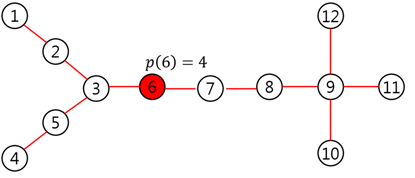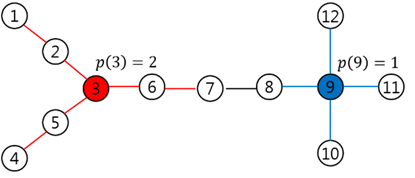시간 제한메모리 제한제출정답맞힌 사람정답 비율
0.5 초 512 MB368664417.460%

## 문제

There is a network of cities where broadcast stations, broadcasting identical information, should be placed on some cities. Each broadcast station has its own transmission power p so that it can broadcast to any city within distance p from it. Here, the distance between two vertices is the number of edges included in the (unique) path between them. In this problem, the network of cities is a tree T with n vertices each of which represents a city.

For a tree T with a set V of n vertices, we will assign a non-negative integer p(v), called a broadcast power, to every vertex v in V such that every vertex u with p(u) = 0 is within distance p(v) from some vertex v with p(v) > 0. Then we can regard the vertices v with p(v) > 0 as broadcast stations of transmission power p(v), and a vertex u with p(u) = 0 can hear the broadcast of v, if u is within distance p(v) from v.

The goal of the problem is to find an assignment of the broadcast powers p(v) of vertices v in V described above, minimizing ∑vVp(v).

For example, in Figure A.1, two cases of an assignment of broadcast powers to vertices are shown. In the case of Figure A.1 (a), only the vertex 6 has the broadcast power 4 and the other vertices have zero. Then all the vertices with the broadcast power 0 can hear the broadcast of the vertex 6. In the case of Figure A.1 (b), two vertices 3 and 9 have the broadcast powers 2 and 1, respectively. Then all the vertices with the broadcast power 0 can hear the broadcast of either the vertex 3 or 9. This case minimizes the sum of broadcast powers.(a) (b)

Figure A.1: Two assignments of broadcast powers

## 입력

Your program is to read from standard input. The first line contains one integer n (1 ≤ n ≤ 5,000) representing the number of vertices of the input tree T. The vertices of T are numbered from 1 to n. Each of the following n-1 lines contains two integers a and b (1 ≤ a, bn) representing an edge to connect two vertices a and b in T.

## 출력

Your program is to write to standard output. Print exactly one line which contains an integer that is the minimum sum of broadcast powers among all the possible assignments of broadcast powers to the vertices in T described above.

## 예제 입력 1

6
1 2
3 2
2 4
5 4
6 4


## 예제 출력 1

2


## 예제 입력 2

12
1 2
2 3
4 5
5 3
3 6
6 7
7 8
8 9
12 9
9 11
10 9


## 예제 출력 2

3